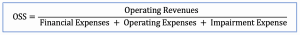# Operational Self Sufficiency

## Definition

Operational self-sufficiency (OSS) is the ratio that is usually used in the Microfinance Institution (MFI) to analyze its ability in generating operating revenues or incomes in order to cover the total cost incurred in running the business.

The operational revenues used in calculating this ratio are the financial incomes the company earned by charging the interest on the loans it provided as well as fee and commission income. Meanwhile, total cost includes operating expenses, financial expenses and impairment expenses on loan loss.

Financial expenses and impairment expenses are included in the calculation because they tend to be a significant amount in this sector. Likewise, the OSS ratio is the type of ratio that focuses on the core revenues and expenses of the business.

A ratio of 100% in OSS would mean a break-even point. The MFI would be doing fine if the ratio is bigger than 100%. On the other hand, if the ratio is less than 100%, it would indicate that MFI is making a loss.

In this case, the MFI may need to either increase operating revenues or decreasing its total costs in order to increase the ratio to a desirable level.

## Operational Self Sufficiency Formula

Operational self-sufficiency ratio can be calculated using the formula as below:• Operating revenues include interest, fee and commission income
• Operating expenses include personnel expense and administrative expense

## Operational Self Sufficiency Example

For example, we have the 2018 income statement of ABC MFI Ltd. as in the table below.

What is the operational self-sufficiency ratio of ABC MFI?

ABC MFI Ltd.
INCOME STATEMENT 2018
Financial Income         2,710,000
Interest income         2,500,000
Fee income            160,000
Commission income              50,000
Financial Expenses          1,340,000
Interest expenses         1,300,000
Fee expenses              40,000
Financial Margin         1,370,000
Impairment expense            140,000
Operating Expenses             650,000
Personnel Expenses            500,000
Net Profit Before Tax             580,000
Tax on Profit expense              82,000
Net Profit After Tax            498,000

## Calculate Operational Self Sufficiency

With the financial information in the income statement above, we can get:

• Operating revenues = financial income = 2,710,000
• Financial expenses = 1,340,000
• Impairment expense = 140,000
• Operating expense = 650,000

Hence, we can calculate operational self sufficiency as below:

OSS ratio = 2,710,000 / (1,340,000 + 140,000 + 650,000) = 127.23%

So the operational self-sufficiency ratio is 127 % which means that the company is doing well to earn sufficient operational revenues to cover its total cost.